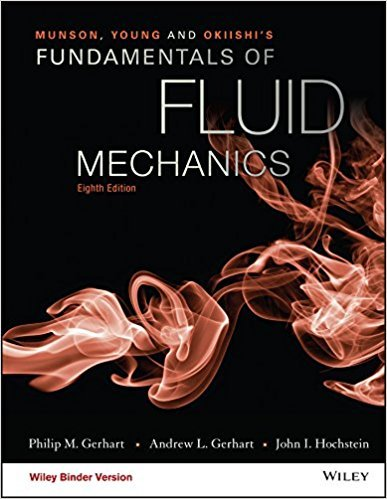×
Get Full Access to Fundamentals Of Fluid Mechanics - 8 Edition - Chapter 4.2.1 - Problem 4.57
Get Full Access to Fundamentals Of Fluid Mechanics - 8 Edition - Chapter 4.2.1 - Problem 4.57

×

# The following pressures for the air flow in 4.24were measured: x = 0 x = 10 m x = 20 mtISBN: 9781119080701 456

## Solution for problem 4.57 Chapter 4.2.1

Fundamentals of Fluid Mechanics | 8th Edition

• Textbook Solutions
• 2901 Step-by-step solutions solved by professors and subject experts
• Get 24/7 help from StudySoup virtual teaching assistantsFundamentals of Fluid Mechanics | 8th Edition

4 5 1 250 Reviews
28
5
Problem 4.57

The following pressures for the air flow in 4.24were measured: x = 0 x = 10 m x = 20 mt = 0 s p = 101 kPa p = 101 kPa p = 101 kPat = 1.0 s p = 121 kPa p = 116 kPa p = 111 kPat = 2.0 s p = 141 kPa p = 131 kPa p = 121 kPat = 3.0 s p = 171 kPa p = 151 kPa p = 131 kPa.Find the local rate of change of pressure p/t and the convectiverate of change of pressure V p/x at t = 2.0 s and x = 10 m.

Step-by-Step Solution:
Step 1 of 3

Oceanography Week 4 The sea surface height varies by surface temperature Warmer water expands, causing it to be slightly elevated­ this has been measured by satellite imaging. Because of this, local sea surface height is a function of surface temperature El Nino runs along the equator and warms the water, changing US climate: warmer winters, droughts in California and the Southwestern corner...

Step 2 of 3

Step 3 of 3

##### ISBN: 9781119080701

The full step-by-step solution to problem: 4.57 from chapter: 4.2.1 was answered by , our top Science solution expert on 03/16/18, 03:21PM. Fundamentals of Fluid Mechanics was written by and is associated to the ISBN: 9781119080701. This full solution covers the following key subjects: . This expansive textbook survival guide covers 112 chapters, and 1357 solutions. This textbook survival guide was created for the textbook: Fundamentals of Fluid Mechanics, edition: 8. Since the solution to 4.57 from 4.2.1 chapter was answered, more than 216 students have viewed the full step-by-step answer. The answer to “The following pressures for the air flow in 4.24were measured: x = 0 x = 10 m x = 20 mt = 0 s p = 101 kPa p = 101 kPa p = 101 kPat = 1.0 s p = 121 kPa p = 116 kPa p = 111 kPat = 2.0 s p = 141 kPa p = 131 kPa p = 121 kPat = 3.0 s p = 171 kPa p = 151 kPa p = 131 kPa.Find the local rate of change of pressure p/t and the convectiverate of change of pressure V p/x at t = 2.0 s and x = 10 m.” is broken down into a number of easy to follow steps, and 108 words.

Unlock Textbook Solution